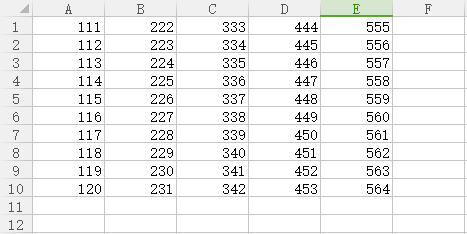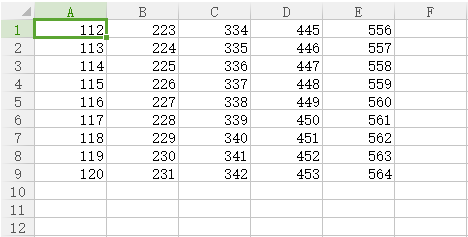python如何删除一个excel文件第5列
``````# coding:utf-8
import pandas
from pandas import DataFrame
data.drop(555,axis = 1)

#sheet_name：返回指定的sheet，如果将sheet_name指定为None，则返回全表，如果需要返回多个表，可以将sheet_name指定为一个列表，例如['sheet1', 'sheet2']
# 删除gender列，需要指定axis为1，当删除行时，axis为0
``````2个回答

del data['E']lasoup 回复lasoup: 列
11 个月之前 回复lasoup 刚又试了，把data.drop(555,axis = 1)替换为del data成功删除第五行
11 个月之前 回复lasoup import pandas from pandas import DataFrame data = pandas.read_excel(r".\aa.xlsx",sheet_name="Sheet1", header=0) data.drop(555,axis = 1) DataFrame(data).to_excel('bb.xlsx', sheet_name='Sheet1', index=False, header=False)
11 个月之前 回复

``````labels = labels.drop(行号, axis = 0)
labels
``````